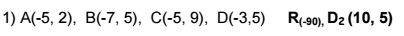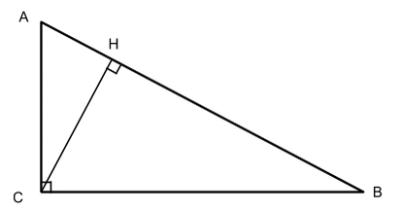# Similarity Unit

This unit is the Similarity Unit being taught by some teachers at Rodriguez High School. It covers clusters:
• G-SRT.A Understand similarity in terms of similarity transformations.
• G-SRT.B Prove theorems involving similarity.
• G-GPE.B Use coordinates to prove simple geometric theorems algebraically.
• G-GMD.B Visualize relationships between two-dimensional and three-dimensional objects.

### Activity 1: (approx 30-40 minutes)

Worksheets: G-SRT.A.2 Similarity Investigation
The first activity should be the investigation of similar triangles. Students measure the sides and angles of five different triangles and record their data in a data table. Four of the five triangles are similar and the last one is not. They are then asked to look for patterns in the data table. The should be able to see that all of the angles are the same except for one.

The next table asks them to calculate the proportion of the sides compared to the first triangle and look for patterns. Again they should see that the proportions are the same except for one triangle.

Using that information, they can write a definition for similar triangles:
Similar triangles are triangles that have corresponding angles that are congruent and corresponding sides that are proportional.
They then practice using the similar "~" symbol in naming similar triangles.

### Activity 2:  (approx 20 minutes)

Worksheets: G-CO.A.5 Similarity Transformations- Dilations (pages 3-4)

This activity has students dilate shapes using a scale factor and a center point. Student should plot the center point and draw a ray to each vertex of the shape. Then take the scale factor and multiply it by the length of the ray to find the new vertex. Repeat for each vertex and connect them to make the dilated shape.

Then they do the process in reverse to determine if the shape in similar or not. They draw a ray that passes through the each corresponding vertex of the shape. If the rays intersect at one point, the shape is similar. They can then determine the scale factor from the length of the rays.

### Pre/Post Assessment

The pre/post assessment can be given at any time. I found it helpful to give the day after lesson 1, so students may have some familiarity with a dilation. There are many ways to complete the task, so the explanation is the focus.
I scanned some of my students work in and had the whole class read and grade them at a later time. This is the rubric we used, and these are the samples that we grades.

### Activity 3: (approx 25 minutes)

Students are asked to determine if triangles are similar based on the measure of their angles, or the ratio of their sides. The are not to actually measure them, but deduce the angles measurements. Focus on using the notation correctly to signify corresponding sides and angles.

### Activity 4: (approx 25 minutes)

We found students need more practice with dilations and determining with the transformation was a dilation from Activity 2. This is more practice.

### Activity 5: (approx 40 minutes)

Students practice transformations by plotting the vertices of polygons and performing the given transformations.### Activity 6: (approx 60-90 minutes)

Application of similar triangles. Students learn how to set up and solve proportions for a missing side.

### Activity 7: (approx 30 minutes)

We found a great opportunity to bring back quadratic equations into the curriculum. This activity has students set up equations using similar triangles, but each equation is quadratic. Solving by completing the square is the fastest, but factoring and the quadratic formula can be used as well. All answers are whole numbers. Some answers will have to be eliminated, e.g., negative lengths of sides.

### Activity 8: (approx 20 minutes)Just what it says. Students justify each step. *This activity needs more development*

### Activity 9: (approx 40 minutes)

Worksheet: Charlie's TestCharlie is a student in a math class and takes a test. Poorly. Student have to be the teacher and grade Charlie and give him/her a feedback. Great review for the test.